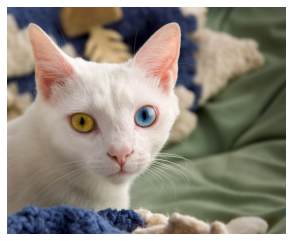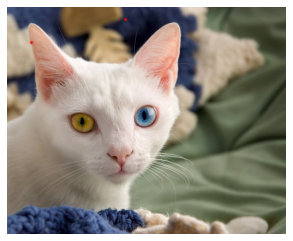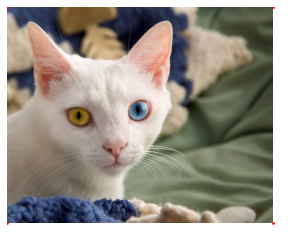Lesson Video:

This article is also a Jupyter Notebook available to be run from the top down. There will be code snippets that you can then run in any environment.

Below are the versions of fastai, fastcore, and wwf currently running at the time of writing this:

• fastai: 2.1.10
• fastcore: 1.3.13
• wwf: 0.0.5

A walk with the Internal API

• What is a PILImage
• How does it work?
• What are these "blocks" and how do they relate?

Today we will go over an example with ImageBlock and PointBlock

Let's import the library:

from fastai.vision.all import *

Below you will find the exact imports for everything we use today

from numpy import ndarray

from fastcore.basics import patch
from fastcore.meta import BypassNewMeta
from fastcore.transform import Transform
from fastcore.xtras import Path

from fastai.data.block import TransformBlock
from fastai.data.transforms import IntToFloatTensor

from fastai.metrics import MSELossFlat

from fastai.torch_core import Tensor, tensor # @patch'd torch.tensor functions

from fastai.vision.data import Image, ImageBlock, PILBase, PointScaler, TensorBase, TensorImageBase, TensorPoint, show_image

We'll use a cat image

What is PILImage? Let's look at the code

class PILImage(PILBase): pass

Okay.. that does nothing. Where do I go from here? We inherit from PILBase, let's try that!

class PILBase(Image.Image, metaclass=BypassNewMeta):
_bypass_type=Image.Image
_show_args = {'cmap':'viridis'}
_open_args = {'mode': 'RGB'}
@classmethod
def create(cls, fn:(Path,str,Tensor,ndarray,bytes), **kwargs)->None:
"Open an Image from path fn"
if isinstance(fn,Tensor): fn = fn.numpy()
if isinstance(fn,ndarray): return cls(Image.fromarray(fn))
if isinstance(fn,bytes): fn = io.BytesIO(fn)

def show(self, ctx=None, **kwargs):
"Show image using merge(self._show_args, kwargs)"
return show_image(self, ctx=ctx, **merge(self._show_args, kwargs))

That looks better. What all does this mean?

Image.Image??

Image.Image means a PIL based image is inherited

Any time we have a datatype we want to use, we need a create and show function. create prepares the file for converting to a tensor, etc. show is our show method.

im = PILImage.create('cat.jpg')
im.show()
<matplotlib.axes._subplots.AxesSubplot at 0x7fd202dedac8>So what have we learned? Each item type needs a create and a show method. How does this relate to ImageBlock?

ImageBlock??
def ImageBlock(cls=PILImage): return TransformBlock(type_tfms=cls.create,
batch_tfms=IntToFloatTensor)

Now we're getting somewhere. If we want to use the DataBlock, each inherit from a TransformBlock

block = TransformBlock(type_tfms=PILImage.create, batch_tfms=IntToFloatTensor)

How would this convert over to a non-image? Let's look at a simple verion, points!

Points

If we take a look at the PointBlock object, we see the following:

PointBlock = TransformBlock(type_tfms=TensorPoint.create, item_tfms=PointScaler)

So let's break this down into two parts, the TensorPoint and the PointScaler

TensorPoint

The goal of the TensorPoint is to turn a list of points into a tensor that we can work with, that is it. Nothing about transforms, just generating some form of a raw input. Let's try building our own based on what we saw earlier, and then see how close we got to the source code

class myTensorPoint(TensorBase):
@classmethod
def create(cls, t):
return cls(tensor(t).view(-1,2).float())

Awesome. Let's try to improve it a bit more. We also want to be careful about our image size, as we may need it for when we transform our image, etc. So let's pass this to it

class myTensorPoint(TensorBase):
@classmethod
def create(cls, t, img_size=None)->None:
return cls(tensor(t).view(-1,2).float(), img_size=img_size)

Let's try it

im.shape
(1927, 2370)
pnts = [[1000,100], [200,300]]
tps = myTensorPoint.create(pnts)
tps
myTensorPoint([[1000.,  100.],
[ 200.,  300.]])

Awesome. Now we need a show method. We'll use a scatter plot as we are dealing with points

class myTensorPoint(TensorBase):
_show_args = dict(s=10, marker='.', c='r')
@classmethod
def create(cls, t, img_size=None)->None:
return cls(tensor(t).view(-1,2).float(), img_size=img_size)

def show(self, ctx=None, **kwargs):
if 'figsize' in kwargs: del kwargs['figsize']
x = self.view(-1,2)
ctx.scatter(x[:,0], x[:,1], **{**self._show_args, **kwargs})
return ctx

Let's try this

tps = myTensorPoint.create(pnts)
tps.show()
---------------------------------------------------------------------------
AttributeError                            Traceback (most recent call last)
<ipython-input-25-a391cc3be866> in <module>()
----> 1 tps.show()

<ipython-input-23-8c86fbd19cec> in show(self, ctx, **kwargs)
8     if 'figsize' in kwargs: del kwargs['figsize']
9     x = self.view(-1,2)
---> 10     ctx.scatter(x[:,0], x[:,1], **{**self._show_args, **kwargs})
11     return ctx

AttributeError: 'NoneType' object has no attribute 'scatter'

Hmmm. Why does this not work? Well we want to overlay it on our image! Let's try passing in an image too

ctx = im.show()
tps.show(ctx=ctx)
<matplotlib.axes._subplots.AxesSubplot at 0x7fd20290b978>Now we see them!

Now there's a few other bits that we want to do. Let's first make our myTensorPoint.create into a Tranform, to allow for what's called setups, we will see more on this later

Transform??
myTensorPointCreate = Transform(myTensorPoint.create)

Any time we deal with these points, we want a loss function of MSELossFlat, lets do this by default (so cnn_learner knows which loss function to use!)

myTensorPointCreate.loss_func = MSELossFlat()

And now let's replace our original myTensorPoint's create function with this new one

myTensorPoint.create = myTensorPointCreate

How close were we to the source code?

class TensorPoint(TensorBase):
"Basic type for points in an image"
_show_args = dict(s=10, marker='.', c='r')

@classmethod
def create(cls, t, img_size=None)->None:
"Convert an array or a list of points t to a Tensor"
return cls(tensor(t).view(-1, 2).float(), img_size=img_size)

def show(self, ctx=None, **kwargs):
if 'figsize' in kwargs: del kwargs['figsize']
x = self.view(-1,2)
ctx.scatter(x[:, 0], x[:, 1], **{**self._show_args, **kwargs})
return ctx

TensorPointCreate = Transform(TensorPoint.create)
TensorPointCreate.loss_func = MSELossFlat()
TensorPoint.create = TensorPointCreate

So now we have seen how to create an item type, and what is needed. Now how do I make sure I deal with the transforms? For instance with keypoints, I need to scale the image and warp it depending on the transforms (such as cropping)

PointScaler

PointBlock = TransformBlock(type_tfms=TensorPoint.create, item_tfms=PointScaler)

PointScaler is an item transform, which means it occurs each time we call the particular item, not when it is turned into a batch. Which is what we need to have happen, as we crop our images during an item transform!

Now how do we deal with this? Transforms have the following:

• order - when does it occur? The lower the value, the sooner it is done
• setups - When we prepare our data, such as our data.c?
• encodes - When we are transforming our image
• decodes - When we are decoding our image

Let's walk through the start of PointScaler. We want it to scale our points and possibly operate differently if we pass in y then x instead of x,y

class myPointScaler(Transform):
order = 1 # Want this to occur first!
def __init__(self, do_scale=True, y_first=False):
self.do_scale, self.y_first = do_scale, y_first

Now let's grab some setups. We want this to take the total available points in our dataset, we can use .numel() to do this

tps.numel()
4
class myPointScaler(Transform):
order = 1 # Want this to occur first!
def __init__(self, do_scale=True, y_first=False):
self.do_scale, self.y_first = do_scale, y_first

def setups(self, dl):
its = dl.do_item(0)
for t in its:
if isinstance(t, TensorPoint): self.c = t.numel()

Where do I go from here? encodes and decodes work by seeing if x follows a type, and if so we perform it. For our input, we want to see what the current size is of our points. Let's first make a method to get the size

class myPointScaler(Transform):
order = 1 # Want this to occur first!
def __init__(self, do_scale=True, y_first=False):
self.do_scale, self.y_first = do_scale, y_first

def setups(self, dl):
its = dl.do_item(0)
for t in its:
if isinstance(t, TensorPoint): self.c = t.numel()

def _grab_sz(self, x):
self.sz = [x.shape[-1], x.shape[-2]] if isinstance(x, Tensor) else x.size

Now let's make an encodes and decodes which just grabs the shape of our points if we have an image

class myPointScaler(Transform):
order = 1 # Want this to occur first!
def __init__(self, do_scale=True, y_first=False):
self.do_scale, self.y_first = do_scale, y_first

def setups(self, dl):
its = dl.do_item(0)
for t in its:
if isinstance(t, TensorPoint): self.c = t.numel()

def _grab_sz(self, x):
self.sz = [x.shape[-1], x.shape[-2]] if isinstance(x, Tensor) else x.size

def encodes(self, x:(PILBase, TensorImageBase)): return self._grab_sz(x)
def decodes(self, x:(PILBase, TensorImageBase)): return self._grab_sz(x)

Now let's make a new one that should either scale or unscale our points based on a transformation. We'll make a simple scale_pnts function to scale them together

def _myScale_pnts(y, sz, do_scale=True, y_first=False):
if y_first: y = y.flip(1)
res = y * 2/tensor(sz).float() -1 if do_scale else y
return TensorPoint(res, img_sz=sz)

Does this work? Let's try

tps
myTensorPoint([[1000.,  100.],
[ 200.,  300.]])
_myScale_pnts(tps, 224)
TensorPoint([[ 7.9286, -0.1071],
[ 0.7857,  1.6786]])

What would a point at the end of an image look like?

im.shape
(1927, 2370)
pnts = [[0,0], [2370,0], [0,1927], [2370, 1927]]
tps = TensorPoint.create(pnts)
tps
TensorPoint([[   0.,    0.],
[2370.,    0.],
[   0., 1927.],
[2370., 1927.]])
ax = im.show()
tps.show(ctx=ax)
<matplotlib.axes._subplots.AxesSubplot at 0x7fd202780d30>s_pnts = [_myScale_pnts(tp, 224) for tp in tps]
s_pnts
[TensorPoint([-1., -1.]),
TensorPoint([20.1607, -1.0000]),
TensorPoint([-1.0000, 16.2054]),
TensorPoint([20.1607, 16.2054])]

Next question: does this hold for other images and image sizes?

url2 = 'https://geekologie.com/2019/08/28/crazy-maine-coon-cat.jpg'
im2 = PILImage.create('cat2.jpg')
im2.shape
(770, 640)
pnts2 = [[0,0], [640,0], [0,770], [640, 770]]
tps2 = TensorPoint.create(pnts2)
ax = im2.show()
tps2.show(ctx=ax)
<matplotlib.axes._subplots.AxesSubplot at 0x7fd2022a7470>[_myScale_pnts(tp, 224) for tp in tps2]
[TensorPoint([-1., -1.]),
TensorPoint([ 4.7143, -1.0000]),
TensorPoint([-1.0000,  5.8750]),
TensorPoint([4.7143, 5.8750])]

We can see that (0,0) is always -1,-1

Now we need a way to undo this.

def _myUnscale_pnts(y, sz): return TensorPoint((y+1)*tensor(sz).float()/2, img_size=sz)
s_pnts
[TensorPoint([-1., -1.]),
TensorPoint([20.1607, -1.0000]),
TensorPoint([-1.0000, 16.2054]),
TensorPoint([20.1607, 16.2054])]

We pass in what the tranformed size is, and we get back our original points

[_myUnscale_pnts(tp, 224) for tp in s_pnts]
[TensorPoint([0., 0.]),
TensorPoint([2370.,    0.]),
TensorPoint([   0., 1927.]),
TensorPoint([2370., 1927.])]

And that's it! We transform our points based on a new image size, and then have it be cropped, rotated, etc

def _scale_pnts(y, sz, do_scale=True, y_first=False):
if y_first: y = y.flip(1)
res = y * 2/tensor(sz).float() - 1 if do_scale else y
return TensorPoint(res, img_size=sz)

def _unscale_pnts(y, sz): return TensorPoint((y+1) * tensor(sz).float()/2, img_size=sz)

class PointScaler(Transform):
"Scale a tensor representing points"
order = 1
def __init__(self, do_scale=True, y_first=False): self.do_scale,self.y_first = do_scale,y_first
def _grab_sz(self, x):
self.sz = [x.shape[-1], x.shape[-2]] if isinstance(x, Tensor) else x.size
return x

def _get_sz(self, x):
sz = x.get_meta('img_size')
assert sz is not None or self.sz is not None, "Size could not be inferred, pass it in the init of your TensorPoint with img_size=..."
return self.sz if sz is None else sz

def setups(self, dl):
its = dl.do_item(0)
for t in its:
if isinstance(t, TensorPoint): self.c = t.numel()

def encodes(self, x:(PILBase,TensorImageBase)): return self._grab_sz(x)
def decodes(self, x:(PILBase,TensorImageBase)): return self._grab_sz(x)

def encodes(self, x:TensorPoint): return _scale_pnts(x, self._get_sz(x), self.do_scale, self.y_first)
def decodes(self, x:TensorPoint): return _unscale_pnts(x.view(-1, 2), self._get_sz(x))

Now how do we make a Block with our new bits?

myPointBlock = TransformBlock(type_tfms=myTensorPoint.create, item_tfms=myPointScaler)

From there, if we want to generate a transform, we @patch it. For example, flip_lr

def _neg_axis(x, axis):
x[...,axis] = -x[...,axis]
return x
@patch
def flip_lr(x:TensorPoint): return TensorPoint(_neg_axis(x.clone(), 0))